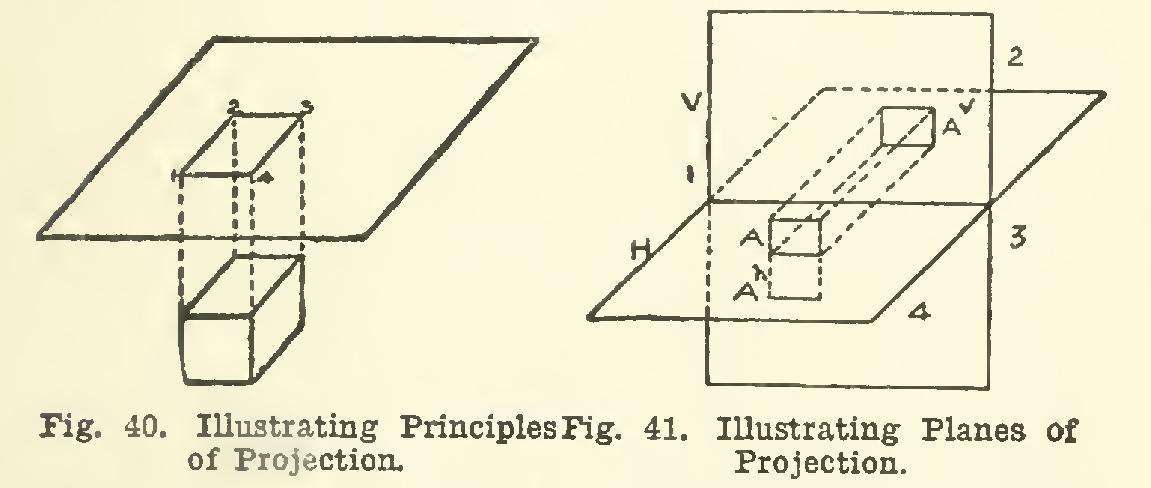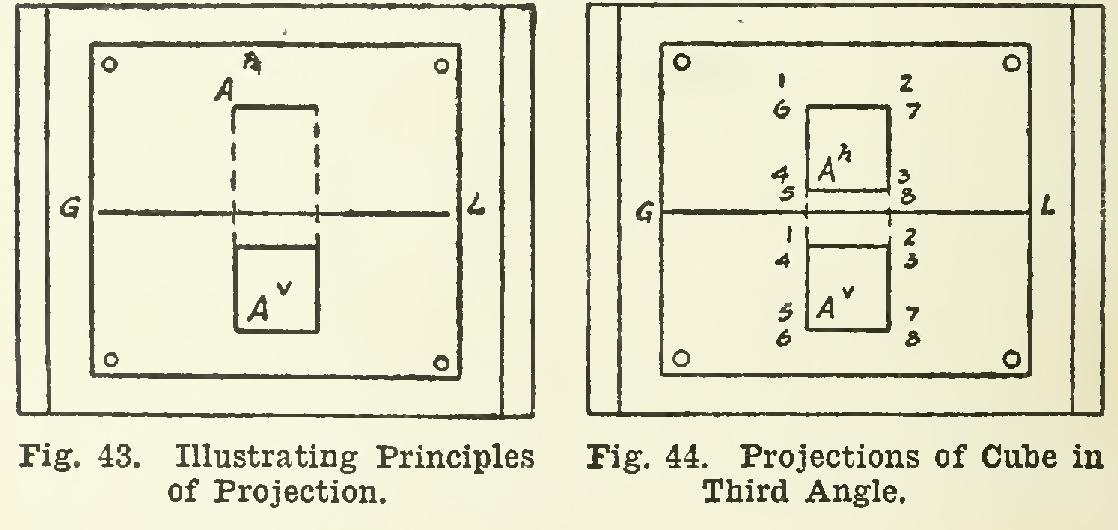Projection 49

plane, cube, plan, square, planes and object

Page: 1 2 3 4 5 6 7 8 9

PROJECTION 49. The term projection as used in mechan ical drafting, may be explained as follows: Assume any object, as a cube, and a plane above it and parallel to the upper face, as in Fig. 40. Now, if parallel lines be drawn from the corners of the cube, and perpendicular to the plane, these perpendiculars will meet the plane in the points 1, 2, 3, and 4; and lines join ing these points will give the four-sided figure 1-2-3-4, which is called the projection of the cube upon the plane. The projection, then, of the cube made in this way, is simply a square equal in size to one of the faces of the cube.There are various kinds of projection, as orthographic or orthogonal, oblique, isometric, axonometric, etc.; but the first is of most prac ti(!al value, and will receive the principal con sideration. Where the simple term "projec tion" is used, orthographic will be understood.

Orthographic Projection While projections may be made on any planes, those most commonly used are one vertical and the other horizontal in position, being thus at right angles. The line of inter section of the two planes is called the ground line, or GL. These planes are known as planes of projection or co-ordinate planes, and for brevity may he referred to as V and H. The position of the planes is as in Fig. 41.

In this figure, let A represent a square placed parallel to the V plane, and with two of its sides perpendicular to the 11 plane. Then the pro jection on the V plane, a square equal to A. is shown at Av, while the projection of A on the II plane is merely one line, Ah.It will be seen that four angles are formed by the intersection of the two planes. These angles, or quadrants, are distinguished as first, second, third, and fourth, as indicated by the numbers, and are always in this same order. For practical purposes, drawings are made as if the object were in either the 1st or the 3d angle.

50. Fig. 42 shows a square A in the 3d angle, placed parallel to V, and two edges perpendicu lar to H. It is projected up to H, giving Ah for the H projection; and forward to V, giving A.∞ as the V projection.

51. Plans and Elevations. In practical work, the term plan is used instead of H projection; and an H projection or plan, unless otherwise expressly stated, is always a view of the top or upper side of any object; hence a plan may often be spoken of as a top view.

Similarly, elevation is the usual term for V projection, and means a view of the front side of an object, or sometimes a view of the right or left side. In actual drawing, the H plane is taken as the plane of the paper as it lies on the drawing board; and the V plane, as an imag inary plane vertical in position and directly in front of the draftsman.

It must be noted that when the plan and the elevation of any object are both made on the same sheet of paper, one can be neither to the right nor left of the other; but one must be drawn on the paper vertically over the other.

The drawings of Figs. 40, 41, and 42 are pic torial, and not actual projections.

52. Actual Projections. In Fig. 43, a T-square line GL may represent the V plane as seen from above; and the whole surface of the paper, the H plane. Then any object in the first or the fourth angle would be projected on H on the near side of GL; and any object in either the second or third angle would be pro jected on the further side of GL.

The actual plan and elevation of the square shown pictorially in the preceding figure may now be made. A straight line A.' parallel to the V plane and on the further side of V, will be the H projection or plan of the square; and the square, in its full size below GL, will repre sent the projection on V.Suppose that the plan of a cube placed in the third angle is to be made; and let the cube be placed with the top and bottom level, and the side faces perpendicular to V. Then, in Fig. 44, the plan will be shown as at A', equal to one side of the cube; and the distance between A' and GL is the distance that the cube is behind the vertical plane.

Page: 1 2 3 4 5 6 7 8 9